# OpenGL核心技术之混合技术

CSDN视频网址：http://edu.csdn.net/lecturer/144

alpha通道的，效果如下所示：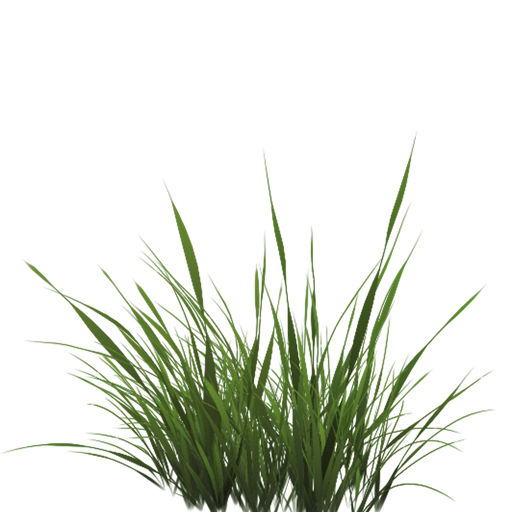unsigned char * image = SOIL_load_image(path, &width, &height, 0, SOIL_LOAD_RGBA);

glTexImage2D(GL_TEXTURE_2D, 0, GL_RGBA, width, height, 0, GL_RGBA, GL_UNSIGNED_BYTE, image);

void main()
{
// color = vec4(vec3(texture(texture1, TexCoords)), 1.0);
color = texture(texture1, TexCoords);
}

vector<glm::vec3> vegetation;
vegetation.push_back(glm::vec3(-1.5f,  0.0f, -0.48f));
vegetation.push_back(glm::vec3( 1.5f,  0.0f,  0.51f));
vegetation.push_back(glm::vec3( 0.0f,  0.0f,  0.7f));
vegetation.push_back(glm::vec3(-0.3f,  0.0f, -2.3f));
vegetation.push_back(glm::vec3( 0.5f,  0.0f, -0.6f));

glBindVertexArray(vegetationVAO);
glBindTexture(GL_TEXTURE_2D, grassTexture);
for(GLuint i = 0; i < vegetation.size(); i++)
{
model = glm::mat4();
model = glm::translate(model, vegetation[i]);
glUniformMatrix4fv(modelLoc, 1, GL_FALSE, glm::value_ptr(model));
glDrawArrays(GL_TRIANGLES, 0, 6);
}
glBindVertexArray(0);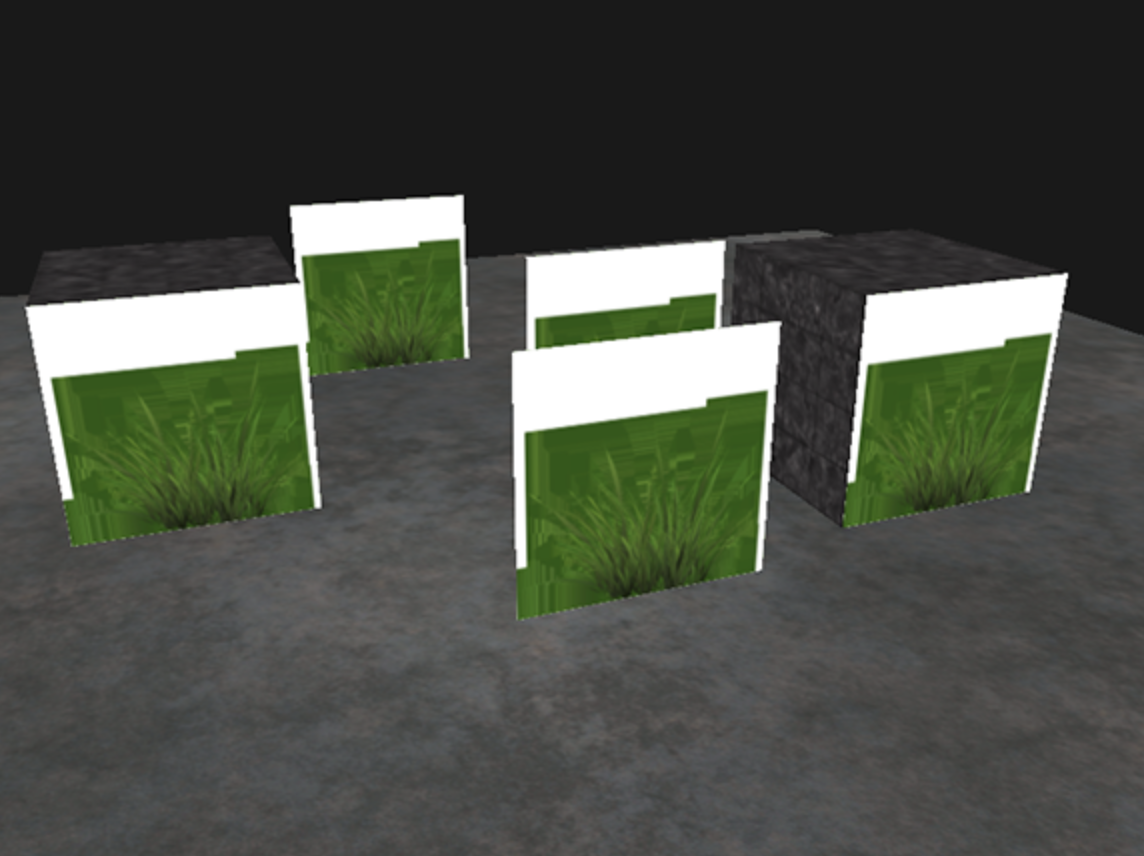#version 330 core
in vec2 TexCoords;

out vec4 color;

uniform sampler2D texture1;

void main()
{
vec4 texColor = texture(texture1, TexCoords);
if(texColor.a < 0.1)
color = texColor;
}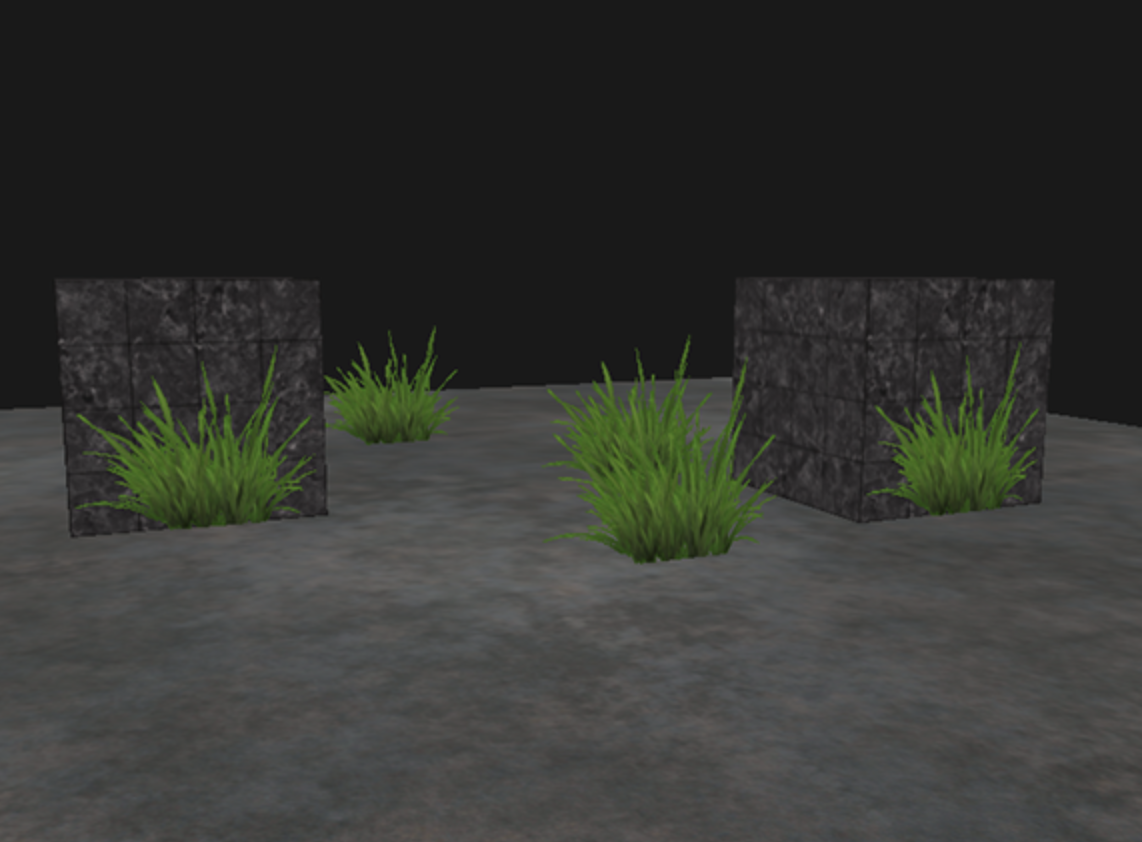#version 330 core
layout (location = 0) in vec3 position;
layout (location = 1) in vec2 texCoords;

out vec2 TexCoords;

uniform mat4 model;
uniform mat4 view;
uniform mat4 projection;

void main()
{
gl_Position = projection * view * model * vec4(position, 1.0f);
TexCoords = texCoords;
}

#version 330 core
in vec2 TexCoords;

out vec4 color;

uniform sampler2D texture1;

void main()
{
vec4 texColor = texture(texture1, TexCoords);
if(texColor.a < 0.1)
color = texColor;
}

glEnable(GL_BLEND);

OpenGL以下面的方程进行混合：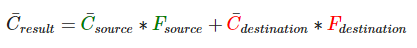• C¯source：源颜色向量。这是来自纹理的本来的颜色向量。
• C¯destination：目标颜色向量。这是储存在颜色缓冲中当前位置的颜色向量。
• Fsource：源因子。设置了对源颜色的alpha值影响。
• Fdestination：目标因子。设置了对目标颜色的alpha影响。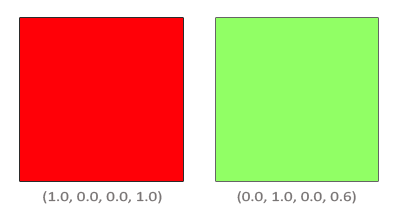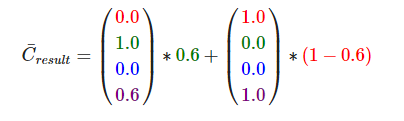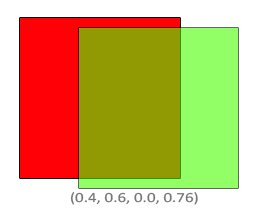void glBlendFunc(GLenum sfactor, GLenum dfactor)接收两个参数，来设置源（source）和目标（destination）因子。OpenGL为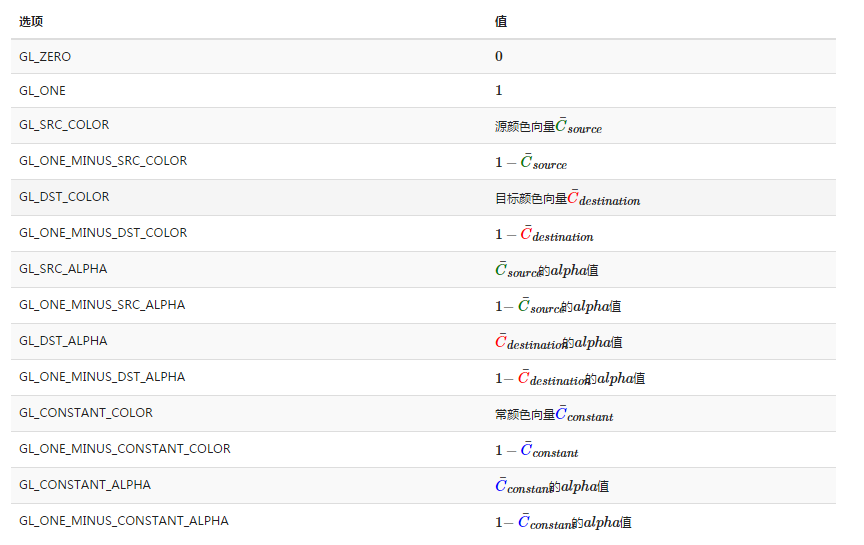glBlendFunc(GL_SRC_ALPHA, GL_ONE_MINUS_SRC_ALPHA);

glBlendFuncSeperate(GL_SRC_ALPHA, GL_ONE_MINUS_SRC_ALPHA,GL_ONE, GL_ZERO);

OpenGL给了我们更多的自由，我们可以改变方程源和目标部分的操作符。现在，源和目标元素已经相加了。如果我们愿意的话，

void glBlendEquation(GLenum mode)允许我们设置这个操作，有3种可行的选项：

• GL_FUNC_ADD：默认的，彼此元素相加：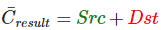• GL_FUNC_SUBTRACT：彼此元素相减：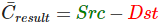• GL_FUNC_REVERSE_SUBTRACT：彼此元素相减，但顺序相反：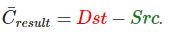glEnable(GL_BLEND);
glBlendFunc(GL_SRC_ALPHA, GL_ONE_MINUS_SRC_ALPHA);

#version 330 core
in vec2 TexCoords;

out vec4 color;

uniform sampler2D texture1;

void main()
{
color = texture(texture1, TexCoords);
}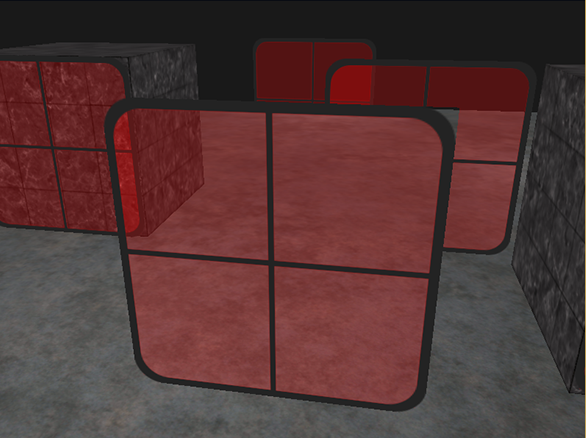std::map<float, glm::vec3> sorted;
for (GLuint i = 0; i < windows.size(); i++) // windows contains all window positions
{
GLfloat distance = glm::length(camera.Position - windows[i]);
sorted[distance] = windows[i];
}

for(std::map<float,glm::vec3>::reverse_iterator it = sorted.rbegin(); it != sorted.rend(); ++it)
{
model = glm::mat4();
model = glm::translate(model, it->second);
glUniformMatrix4fv(modelLoc, 1, GL_FALSE, glm::value_ptr(model));
glDrawArrays(GL_TRIANGLES, 0, 6);
}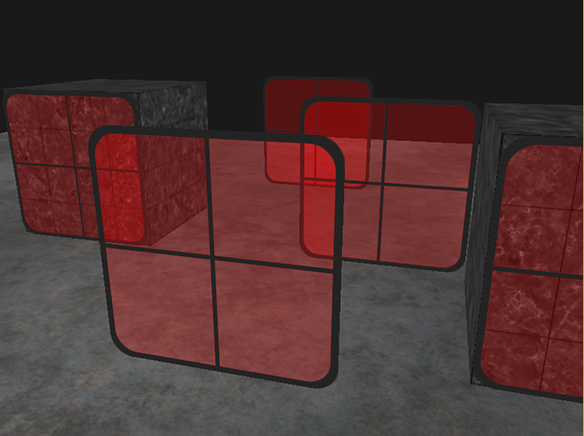先看第一步，我们使用片段着色器来填充链表：

#version 420 core

layout (early_fragment_tests) in;

layout (binding = 0, r32ui) uniform uimage2D head_pointer_image;
layout (binding = 1, rgba32ui) uniform writeonly uimageBuffer list_buffer;

layout (binding = 0, offset = 0) uniform atomic_uint list_counter;

layout (location = 0) out vec4 color;

in vec4 surface_color;

uniform vec3 light_position = vec3(40.0, 20.0, 100.0);

void main(void)
{
uint index;
uvec4 item;

index = atomicCounterIncrement(list_counter);

item.y = packUnorm4x8(surface_color);
item.z = floatBitsToUint(gl_FragCoord.z);
item.w = 255 / 4;

imageStore(list_buffer, int(index), item);

//color = surface_color;
}

#version 330

layout (location = 0) in vec3 position;
layout (location = 1) in vec3 normal;

uniform mat4 model_matrix;
uniform mat4 view_matrix;
uniform mat4 projection_matrix;

uniform float minAlpha = 0.5f;

out vec4 surface_color;

void main(void)
{
vec3 color = normal;
if (color.r < 0) { color.r = -color.r; }
if (color.g < 0) { color.g = -color.g; }
if (color.b < 0) { color.b = -color.b; }
vec3 normalized = normalize(color);
float variance = (normalized.r - normalized.g) * (normalized.r - normalized.g);
variance += (normalized.g - normalized.b) * (normalized.g - normalized.b);
variance += (normalized.b - normalized.r) * (normalized.b - normalized.r);
variance = variance / 2.0f;// range from 0.0f - 1.0f
float a = (0.75f - minAlpha) * (1.0f - variance) + minAlpha;
surface_color = vec4(normalized, a);

gl_Position = projection_matrix * view_matrix * model_matrix * vec4(position, 1.0f);
}

#version 420 core
// The per-pixel image containing the head pointers
layout (binding = 0, r32ui) uniform uimage2D head_pointer_image;
// Buffer containing linked lists of fragments
layout (binding = 1, rgba32ui) uniform uimageBuffer list_buffer;

// This is the output color
layout (location = 0) out vec4 color;

// This is the maximum number of overlapping fragments allowed
#define MAX_FRAGMENTS 40

// Temporary array used for sorting fragments
uvec4 fragment_list[MAX_FRAGMENTS];

void main(void)
{
uint current_index;
uint fragment_count = 0;

while (current_index != 0 && fragment_count < MAX_FRAGMENTS)
{
fragment_list[fragment_count] = fragment;
current_index = fragment.x;
fragment_count++;
}

if (fragment_count > 1)
{
for (uint i = 0; i < fragment_count - 1; i++)
{
uint p = i;
uint depth1 = (fragment_list[p].z);
for (uint j = i + 1; j < fragment_count; j++)
{
uint depth2 = (fragment_list[j].z);
if (depth1 < depth2)
{
p = j; depth1 = depth2;
}
}
if (p != i)
{
uvec4 tmp = fragment_list[p];
fragment_list[p] = fragment_list[i];
fragment_list[i] = tmp;
}
}
}

vec4 final_color = vec4(0.0);

for (uint i = 0; i < fragment_count; i++)
{
vec4 modulator = unpackUnorm4x8(fragment_list[i].y);
//final_color = mix(final_color, modulator, modulator.a);
final_color = final_color * (1.0f - modulator.a) + modulator * modulator.a;
}

color = final_color;
// color = vec4(float(fragment_count) / float(MAX_FRAGMENTS));
}

#version 420 core

in vec3 position;

uniform mat4 model_matrix;
uniform mat4 view_matrix;
uniform mat4 projection_matrix;

void main(void)
{
gl_Position = projection_matrix * view_matrix * model_matrix * vec4(position, 1.0f);
//gl_Position = vec4(position, 1.0f);
}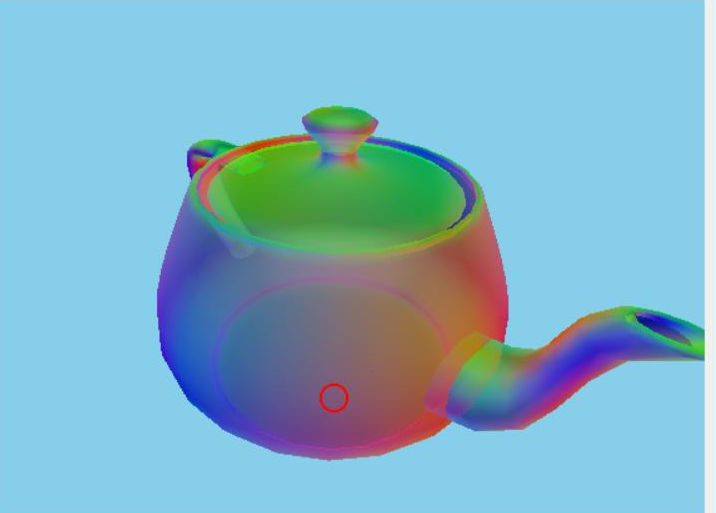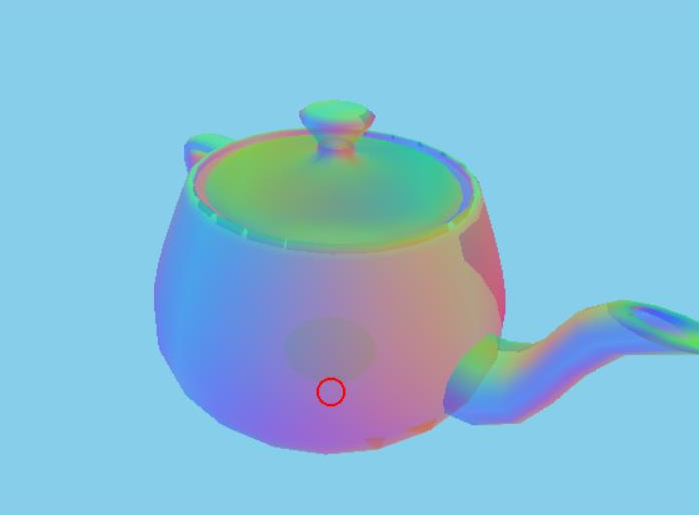©️2019 CSDN 皮肤主题: 大白 设计师: CSDN官方博客